# Thread: Chain Rule

1. ## Chain Rule

f(x) = (5x^3 + 1)^8 (4x^5 + 3)^7

How do I apply the chain rule to a problem like this?

2. Assuming you want to differentiate it, read it as a two-part product, apply the product rule, and each time you do a differentiation for the sake of the product rule you'll need to apply the chain rule - because each of the two parts of the product is a composite. Will put a pic, in a minute.

Just in case a picture helps...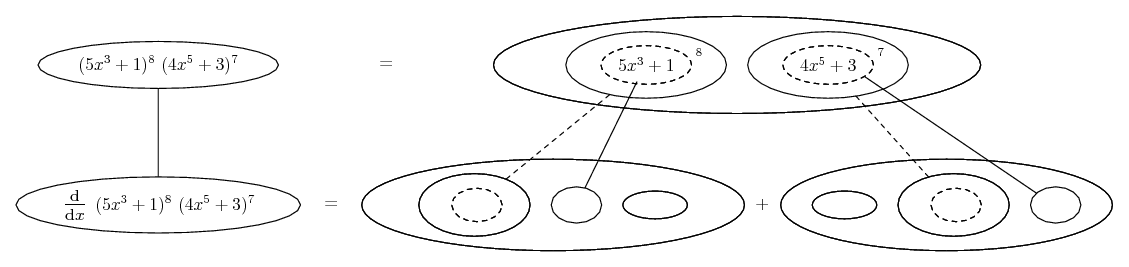... where (key in first spoiler)...

Spoiler:... is the chain rule. Straight continuous lines differentiate downwards (integrate up) with respect to x, and the straight dashed line similarly but with respect to the dashed balloon expression (the inner function of the composite which is subject to the chain rule). This is embedded in the legs-uncrossed version of...... the product rule. Again, straight lines differentiate downwards w.r.t. x.

Spoiler: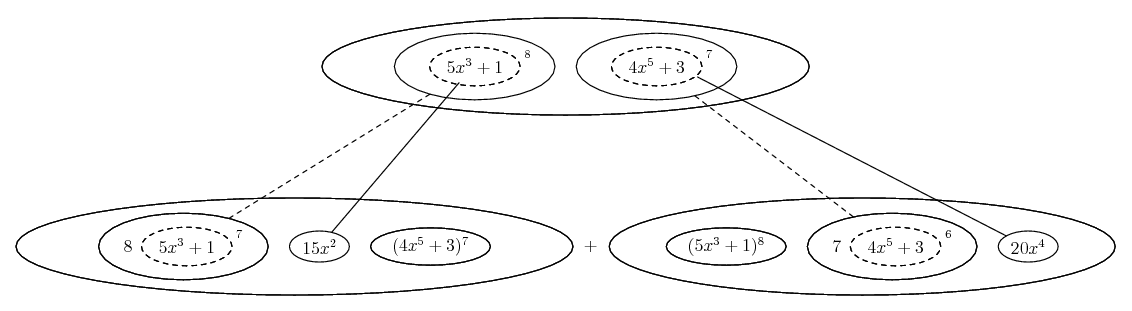... or...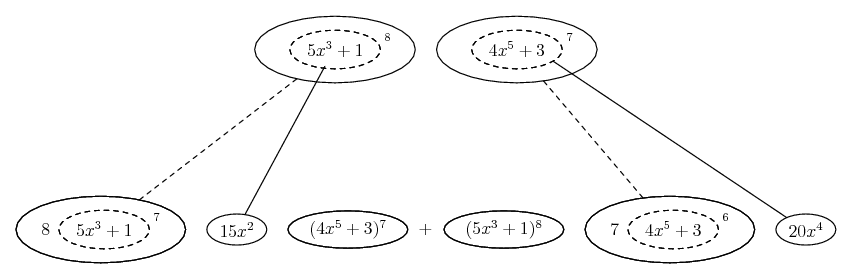... according to taste.

Alternatively, take logs of both sides, then differentiate...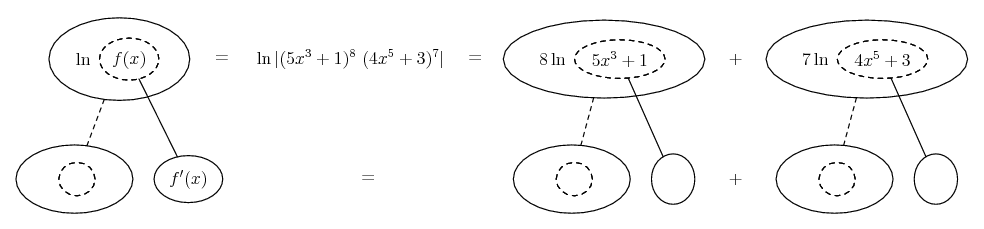Spoiler: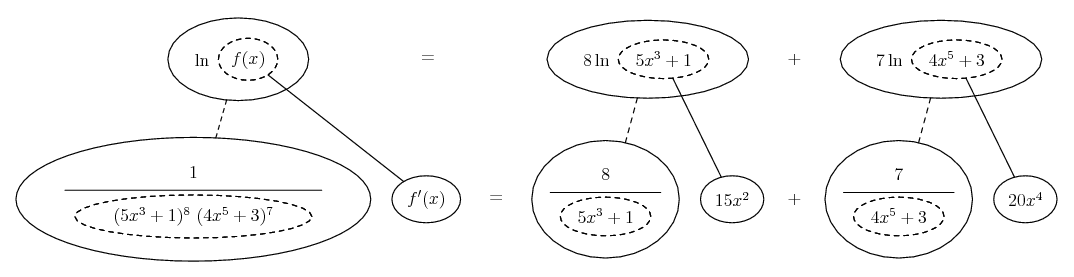Then solve the bottom row for f'(x).

_________________________________________

Don't integrate - balloontegrate!

Balloon Calculus; standard integrals, derivatives and methods

Balloon Calculus Drawing with LaTeX and Asymptote!

3.Originally Posted by bobsanchezf(x) = (5x^3 + 1)^8 (4x^5 + 3)^7

How do I apply the chain rule to a problem like this?
$\displaystyle u=5x^3+1,\ s=u^8=f(u)$

"u" is a function of x, "s" is a function of u.

$\displaystyle v=4x^5+3,\ t=v^7=f(v)$

"v" is a function of x, "t" is a function of v.

$\displaystyle f(x)=st$

$\displaystyle f'(x)=t\frac{ds}{dx}+s\frac{dt}{dx}$

But $\displaystyle s=f(u)$ and $\displaystyle t=f(v)$

hence, applying the Chain Rule...

$\displaystyle f'(x)=t\frac{ds}{du}\frac{du}{dx}+s\frac{dt}{dv}\f rac{dv}{dx}$

#### Search Tags

chain, rule